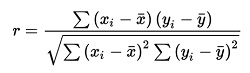# How to perform Correlation Test in Python?

Python Correlation Test: In this tutorial, we will learn about the correlation test and how to perform correlation test in Python? By Shivang Yadav Last updated : September 13, 2023

We have different types of correlation by the simplest way to represent the relationship between two variables quantitatively is Pearson Correlation coefficient.

## Pearson correlation coefficient

Pearson correlation coefficient, denoted as "r" or the Pearson's r, is a statistic that quantifies the linear relationship or association between two continuous variables. It measures the strength and direction of the linear relationship between the variables, ranging from -1 to 1. Here's how it works:

• r = 1, it indicates a perfect positive linear relationship. This means that as one variable increases, the other variable increases proportionally.
• r = -1, it indicates a perfect negative linear relationship. This means that as one variable increases, the other variable decreases proportionally.
• r = 0, it indicates no linear relationship between the variables. They are not correlated in a linear fashion, but there may still be other types of relationships or associations.

The formula for calculating the Pearson correlation coefficient (r) between two variables, X and Y, with n data points, is:Where,

• Xi and Yi are the individual data points.
• X and Y are the means (averages) of X and Y.

## Perform correlation test

Python also provides a function to perform the test. The scipy library contains pearsonr() functions that return two values, the pearson correlation coefficient and p-value.

Syntax

```scipy.stats.pearsonr(x, y)
```

## Python program to perform correlation test

```import numpy as nump
import scipy.stats as scstats

# Creating the numpy arrays
dataset1 = nump.array([3, 4, 4, 5, 7, 8, 10, 12, 13, 15])
dataset2 = nump.array([2, 4, 4, 5, 4, 7, 8, 19, 14, 10])

print(f"The values in dataset1 is \n{dataset1}")
print(f"The values in dataset2 is \n{dataset2}")

# Calculation of pearson correlation coefficient
pearCoeff = scstats.stats.pearsonr(dataset1, dataset2)

print(f"Pearson correlation coefficient is\n{pearCoeff}")
```

Output:

```The values in dataset1 is
[ 3  4  4  5  7  8 10 12 13 15]
The values in dataset2 is
[ 2  4  4  5  4  7  8 19 14 10]
Pearson correlation coefficient is
PearsonRResult(statistic=0.8076177030748631, pvalue=0.004717255828132089)
```